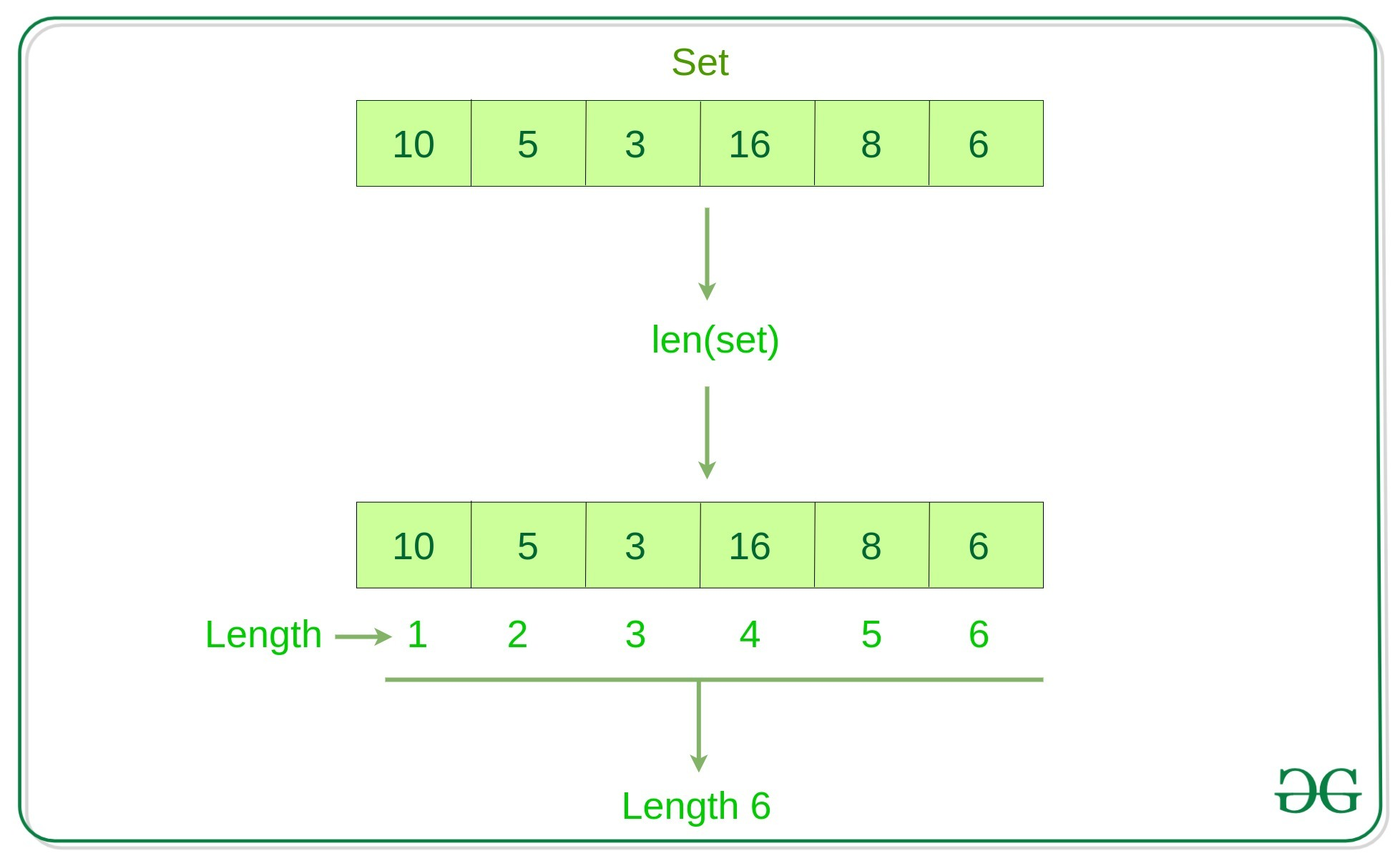Related Articles
Find the length of a set in Python
• Last Updated : 17 May, 2020

In Python, a Set is a collection data type that is unordered and mutable. A set cannot have duplicate elements. Here, the task is to find out the number of elements present in a set. See the below examples.

Examples:

```Input: a = {1, 2, 3, 4, 5, 6}
Output: 6

Input: a = {'Geeks', 'For'}
Output: 2
```

Thee idea is use len() in PythonExample 1:

 `# Python program to find the length ` `# of set ` ` `  `set1 ``=` `set``()   ` `   `  `# Adding element and tuple to the Set  ` `set1.add(``8``)  ` `set1.add(``9``)  ` `set1.add((``6``, ``7``))  ` ` `  `print``(``"The length of set is:"``, ``len``(set1)) `

Output:

```The length of set is: 3
```

Example 2:

 `n ``=` `len``({``1``, ``2``, ``3``, ``4``, ``5``}) ` ` `  `print``(``"The length of set is:"``, n) `

Output:

```The length of set is: 5
```

How does len() work?
`len()` works in `O(1)` time as the set is an object and has a member to store its size. Below is description of `len()` from Python docs.

Return the length (the number of items) of an object. The argument may be a sequence (such as a string, bytes, tuple, list, or range) or a collection (such as a dictionary, set, or frozen set).

Attention geek! Strengthen your foundations with the Python Programming Foundation Course and learn the basics.

To begin with, your interview preparations Enhance your Data Structures concepts with the Python DS Course.

My Personal Notes arrow_drop_up
Recommended Articles
Page :